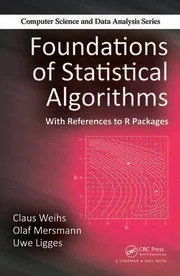Want to share your content on R-bloggers? click here if you have a blog, or here if you don't.There is computational statistics and there is statistical computing. And then there is statistical algorithmic. Not the same thing, by far. This 2014 book by Weihs, Mersman and Ligges, from TU Dortmund, the later being also a member of the R Core team, stands at one end of this wide spectrum of techniques required by modern statistical analysis. In short, it provides the necessary skills to construct statistical algorithms and hence to contribute to statistical computing. And I wish I had the luxury to teach from Foundations of Statistical Algorithms to my graduate students, if only we could afford an extra yearly course…

“Our aim is to enable the reader (…) to quickly understand the main ideas of modern numerical algorithms [rather] than having to memorize the current, and soon to be outdated, set of popular algorithms from computational statistics.”(p.1)

The book is built around the above aim, first presenting the reasons why computers can produce answers different from what we want, using least squares as a mean to check for (in)stability, then second establishing the ground forFishman Monte Carlo methods by discussing (pseudo-)random generation, including MCMC algorithms, before moving in third to bootstrap and resampling techniques, and  concluding with parallelisation and scalability. The text is highly structured, with frequent summaries, a division of chapters all the way down to sub-sub-sub-sections, an R implementation section in each chapter, and a few exercises.

“The well-established practice of testing new algorithms on standard problems does not in any way assess the general capabilities of the algorithm under test.” (p.87)

The first chapter(s) (Chap.2) is(are) are quite similar to intro courses to computer-science, with detailed analyses of sorting algorithms, Turing machines in action, computing with floating-point numbers, and understanding the limited precision of floating-point computation. Chap. 3 details the verification of computer algorithms in the case of linear least square solvers. The central tool there is the condition number, with some dose of matrix algebra some students may find unsavoury. Chap. 4 is called “Iteration” and deals with the equivalent of Chap. 3 when no closed-form analytic solution is available, introducing various techniques of numerical optimisation (Newton, quasi-Newton, gradient, Levenberg-Marquardt, Nelder-Mead, &c.).  The chapter includes a very short introduction to simulated annealing, which unfortunately includes a typo (acknowledged by the authors) since the energy is the reverse of what is should be, t/Δ instead of Δ/t. It also considers the maximisation of a Gaussian mixture likelihood as an example of a non-convex problem, without mentioning it is a problem per se for having an infinite maximum. Chap. 5 examines the theoretical properties of the Partial Least Squares and of the EM algorithms. Even though those algorithms are essential in statistical computing, this chapter feels an an outlier for its specific focus.

“Independence chain MCMC is thus at least as effective as the rejection method.” (p.325)

Chap. 6 returns to a computer-science presentation of randomness, albeit without the common if barren debate about the nature and definition of the thing. Similarly to Fishman (1996), the book spends some space on describing discrete uniform generators in details, in tune with the initial aim even though the conclusion is to “use the code provided by the original authors” (p.279). In my opinion, Fishman (1996) covers this aspect in a more thorough manner, with a deeper focus on approximation errors in the continuous case. And the coverage of the non-uniform standard distributions is quite superficial (if compared with the iconographic Devroye (1985) [do not pay \$600 for a book available on Luc’s page!]). The second part of the chapter is about rejection and MCMC methods. Once again in agreement with its stated goal, the book focus on the convergence of the Gibbs sampler and of Metropolis-Hastings algorithms. However, it stops at the uniform ergodicity case, namely when the transition kernels are uniformly lower-bounded. The chapter concludes with a detailed illustration using BRugs the 2006 R interface to BUGS. Chap. 7 covers both resampling (cross-validation and bootstrap) and machine learning classification (LDA, kNN) and regression (neural net) methods. (Note that JAGS is also mentioned in this chapter, if not STAN.)

“We will restrict ourselves to nodes running Linux or some other Unix-like operating systems.” (p.430)

The terminal Chap. 8 deals with scaling and parallelisation, starting with a fairly lengthy description of the evolution of computer and parallelisation software, followed by a short section on code optimisation and an introduction to parallel programming, incl. the foreach R package. This is illustrated with a k-means algorithm. The conclusion quickly mentions “the upcoming trend of using graphics processing units” (p.440) and “MapReduce [that] brings the function on a node that is close to the data (…) a filesystem or storage layer that distributes massive data sets across the nodes”(p.442). The final conclusion that “while scaling an algorithm is desirable and parallel computing can be fun and rewarding, we should not overdo it” (p.444).  My own conclusion is that this is a rich book that should benefit a specific niche of statistical graduates and would-be-statisticians, namely those ready to engage into serious statistical programming. It should provides them with the necessary background, out of which they should develop their own tools. A possible small niche but upon which the profession’s future depends!

Filed under: Books, Linux, R, Statistics, University life Tagged: big data, GPU, Hadoop, MapReduce, optimisation, parallelisation, R, R package, random number generation, randomness, scalability, simulation, statistical algorithms, statistical computing